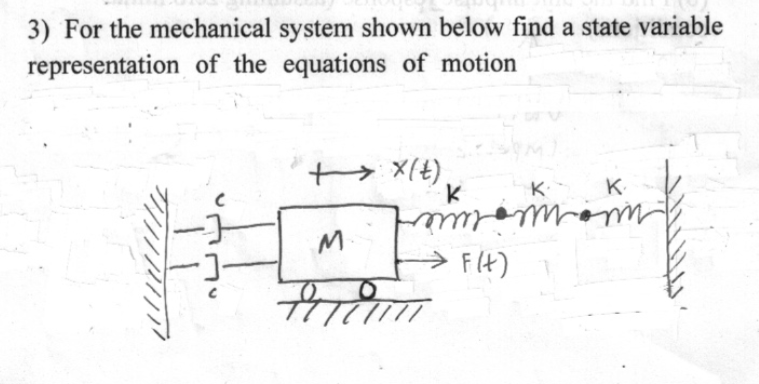Home / Expert Answers / Mechanical Engineering / show-all-work-step-by-step-3-for-the-mechanical-system-shown-below-find-a-state-variable-representa-pa835

# (Solved): Show all work step by step 3) For the mechanical system shown below find a state variable representa ...Show all work step by step

3) For the mechanical system shown below find a state variable representation of the equations of motion

We have an Answer from Expert

==>

To find the state variable representation of the given mechanical system, we need to derive the equations of motion based on the given diagram. From the diagram, it seems that we have a mass-spring-damper system with an external force.

Let's denote the state variables as follows:
x? = position of the mass (displacement)
x? = velocity of the mass

The equation of motion for the mass can be written as:

Where:
m = mass of the system
k?, k? = spring constants
c = damping coefficient
F(t) = external force

To convert this second-order differential equation into a set of first-order differential equations, we introduce two new state variables:
(velocity)
(acceleration)

Now, we can rewrite the equation of motion as a system of first-order differential equations:

Finally, the state variable representation of the system is given by:

We have an Answer from Expert